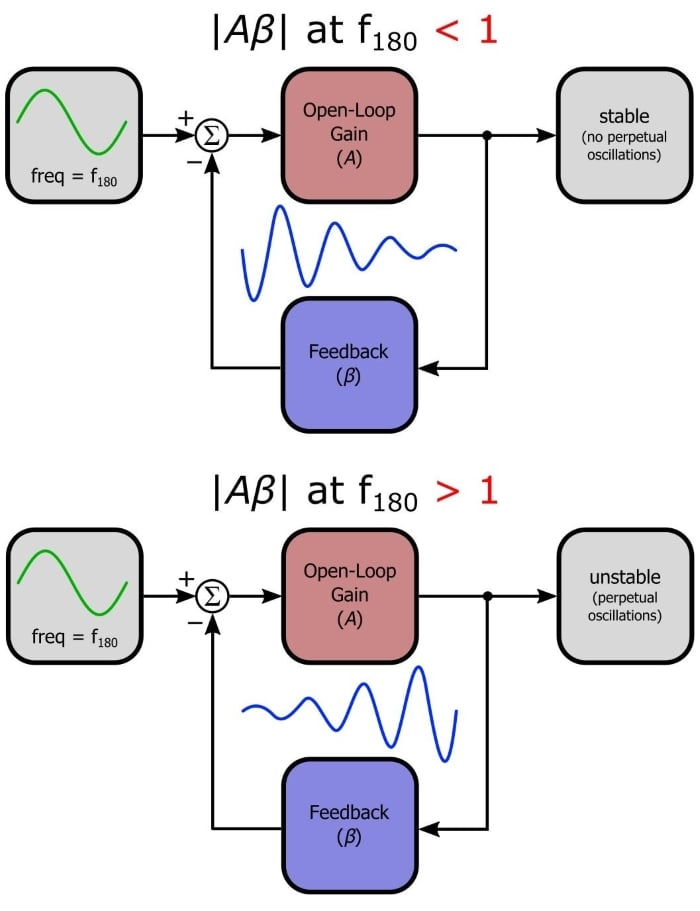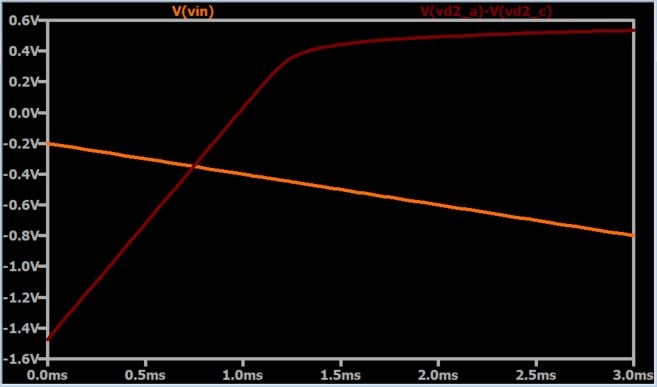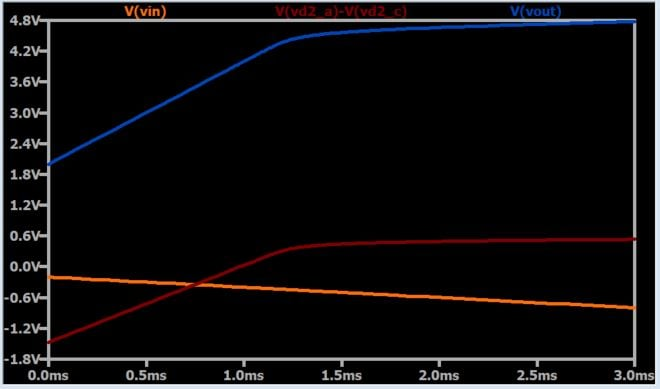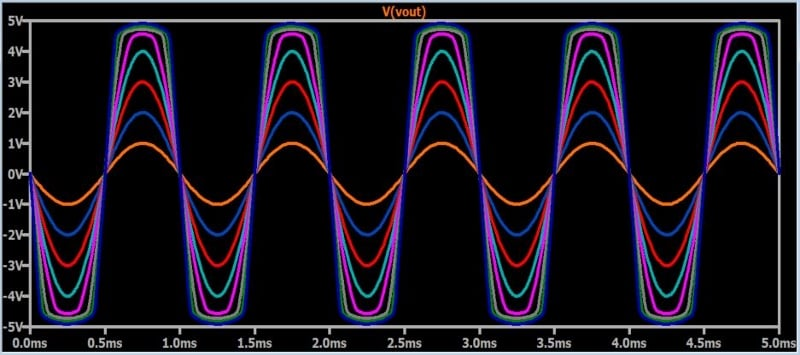Technical Article

# An Op-Amp Limiter: How to Limit the Amplitude of Amplified Signals

January 11, 2018 by Robert Keim

## This article, part of AAC’s Analog Circuit Collection, explores the design and functionality of an op-amp-based limiter.

This article, part of AAC’s Analog Circuit Collection, explores the design and functionality of an op-amp-based limiter.

Two types of amplification are very easy to implement. The first is unity-gain amplification, which requires either a voltage follower or, if no buffering is required, a PCB trace; this approach works wonders when the amplitude of the input signal is more or less within the functional range of the device’s other signal-processing circuitry. The second is fixed-gain amplification, which requires an op-amp and two resistors. If the input signal’s amplitude is fairly constant, or if the downstream circuitry has good dynamic range, the fixed-gain approach might be more than adequate.

There are some situations, however, in which the design requires an amplifier that imposes limitations on the output signal. What I mean is the following: For small input signals, the gain is a certain value. This gain remains more or less unchanged as the input amplitude increases, which is fine—perhaps the downstream circuitry can tolerate a fairly wide range of input amplitudes. But at some point, the amplifier needs to stop amplifying, because the downstream components will perform poorly (or maybe not at all) if the input amplitude exceeds a certain threshold. An amplifier that behaves in this way is called a limiter, because it also limits the output amplitude by incorporating a nonlinear response into its transfer function.

### Applications

The previous paragraph explains the generic application of a limiter. It is basically a more straightforward way of implementing automatic gain control (AGC), though a limiter is not called an AGC circuit and for good reason—AGC uses feedback to ensure that the output signal always has a certain amplitude, whereas a limiter merely ensures that the output doesn’t exceed a certain amplitude. Nonetheless, the general idea is similar: provide an amplified output that, for the entire range of possible input amplitudes, stays within the dynamic range of the downstream circuit. A specific example of this type of application is an RF receiver circuit in which a limiter is used to protect a sensitive low-noise amplifier from unexpected high-amplitude signals.

A less obvious, though still important, application of limiters is found in analog oscillator circuits. If you have read the Negative Feedback series, you know that negative feedback can cause a circuit to become unstable. In other words, with an improperly designed feedback network, a change in the input can lead to output oscillations that increase in magnitude until the amplifier saturates.But what if we want an oscillator rather than an amplifier? Well, it is theoretically possible to create an amplifier that has perfectly marginal stability, such that the output will exhibit sustained oscillations. But this can’t work in real life, because something in the circuit or the external environment will change slightly and “tip the balance,” resulting in oscillations that either diminish toward zero or increase toward saturation. A limiter can be used to overcome this serious impediment to designing a reliable oscillator: the initial balance is intentionally tipped in favor of the increase-toward-saturation scenario, but the limiter reduces the loop gain as amplitude increases, resulting in stable oscillation.

### The Circuit

Here it is:First let’s look at how the circuit works. We’ll just cover the basic concepts here; you can explore the functionality in greater detail via simulations. The nonlinear amplitude control is achieved (not surprisingly) by incorporating the nonlinear current–voltage characteristics of a diode. When the output voltage is low, both diodes are reverse-biased. This means that we can analyze the circuit as if they aren’t even there, and when we do that, we see that the limiter is just a typical inverting op-amp with some resistors connected between the output and the supply voltages. As expected, then, the limiter is just a normal amplifier as long as the output is not high or low enough to provoke its limiting functionality.

As the output increases, though, the voltage at the anode of D2 starts to increase relative to the voltage at the op-amp’s inverting input terminal. Eventually the voltage across the diode will reach ~0.6 V, and the diode will begin to conduct. With D2 conducting, RF is bypassed and the op-amp becomes a voltage follower—in other words, the gain is reduced and the output amplitude is limited. This behavior is shown in the following plot.The input voltage is decreasing, and consequently the output voltage is increasing. The voltage at the anode of D2 is also increasing, and when the input voltage reaches about –0.4 V, the voltage across D2 is high enough to make the diode start conducting. The voltage across D2 then levels out, as we would expect from a forward-biased diode. The next plot confirms that the output voltage also levels out.An analogous process occurs with diode D1.

### Design Details

The non-limiting gain is set using RF and R1; as you can see in the schematic above, my circuit is set for a non-limiting gain of 10. The next task is establishing the positive and negative voltage limits. If you ponder the circuit for a few minutes you will see that R4 and R5 form a voltage divider, such that the D2 anode voltage (and by extension the positive limit voltage) will depend on the ratio of R4 to R5. Similarly, the D1 cathode voltage (and by extension the negative limit voltage) depends on the ratio of R3 to R2. The full equations (courtesy of Microelectronic Circuits) are as follows:

$$negative\ limit=V_{SUPPLY_{NEG}}\frac{R3}{R2}-V_{DIODE}\left(1+\frac{R3}{R2}\right)$$

$$positive\ limit=V_{SUPPLY_{POS}}\frac{R4}{R5}+V_{DIODE}\left(1+\frac{R4}{R5}\right)$$

I calculated resistor values for limits of +5 V and –5 V. The following plot shows that the circuit works as expected; the different traces are the output signals for input-signal amplitudes ranging from 0.1 V to 1.1 V. I really appreciate those smooth transitions from the linear behavior to the limiting behavior.### Conclusion

We discussed the applications and functionality of a straightforward op-amp-based limiter, and we looked at a simple design example. If you want to explore this circuit further or experiment with your own design, feel free to download my LTspice schematic file by clicking on the orange button.

OpAmpLimiter.zip• B
bordonbert January 19, 2018

Clever and ultra simple circuit.  I know its a bit mundane for these hallowed halls but this has the makings of a much better and controllable overdrive/distortion pedal for guitar work.  It simulates beautifully and the clipping action can be made soft or hard as you will.  Excellent article, many thanks.

Like.
• C
chuckdavisdr March 26, 2020

Thanks for this article…really well written and took some work to do! My one comment is just that it looks like the negative supply should have its positive “terminal” connected to ground and the negative side to V2.

Like.
•RK37 March 27, 2020
Thanks for reading, and I'm glad that you liked the article. The voltage source labelled V2 has a value of negative 12 V; that's why I have the positive terminal connected to the circuit and the negative terminal connected to ground.
Like.